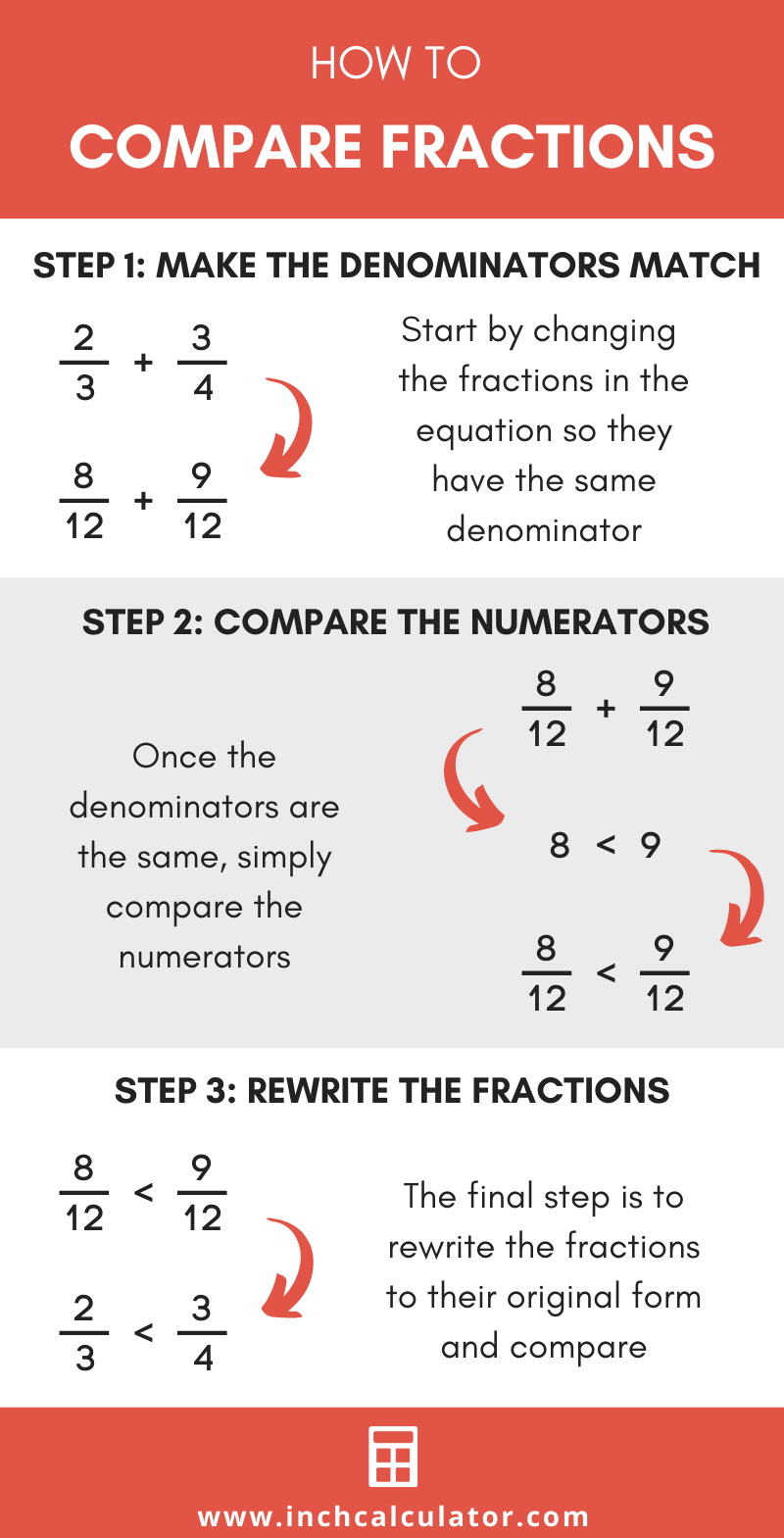# Comparing Fractions Calculator

Compare fractions, decimals, and percentages to find which is greater than, less than, or if they are equal.

Enter fractions, numbers, or percents
?

## Solution:

12 / 3<1.75
12 / 3 is less than 1.75

### Steps to Compare Fractions and Numbers

Step One: convert all numbers to decimal

12 / 3 = 1.6666666666667

1.75 = 1.75

Step Two: compare the decimal values

1.6666666666667 < 1.75

Step Three: rewrite the numbers using the original values

12 / 3 < 1.75
Learn how we calculated this below

## How to Compare Fractions

Comparing fractions might seem challenging; it seems a bit like comparing apples and oranges. Fortunately, there are a few methods for comparing one fraction to another.### Method One: Compare Fractions by Converting to Decimal First

The first way to compare fractions is to convert them to a decimal value. Divide the numerator of each fraction by the denominator, then compare the decimal values.

Here’s a tip: use our fraction to decimal calculator to get the decimal value for your fraction. You might also want to check out our decimal equivalents chart to see the decimal equivalents for common fractions.

### Method Two: Compare Fractions By Finding the Common Denominator

Another way to compare fractions is to rewrite all the fractions to equivalent fractions with the same denominator. To do this, find the greatest common denominator, then make the denominators of each fraction equal.

Start by finding the lowest common denominator, which we sometimes refer to as the least common denominator. This is the smallest number that each denominator divides equally into. If you’re not sure how to do this, our lowest common denominator calculator will help you find it.

For example, let’s find the lowest common denominator of 6 and 9.

6 × 3 = 18
9 × 2 = 18
LCD = 18

Next, divide the lowest common denominator by the denominator of the fraction to find the multiple. Then, multiply the numerator by the multiple to get the new numerator. Put the new numerator over the lowest common denominator to get the new fraction.

For example, convert 1 / 6 to a fraction with a denominator of 18.

18 ÷ 6 = 3
3 × 1 = 3
1 / 6 = 3 / 18

You’ll want to repeat this step for each fraction until they all have the same denominator.

You can also use an equivalent fractions calculator or our equivalent fraction chart to see equivalents for common fractions, which might help shortcut the conversion.

Finally, compare the numerators to find which fraction is the largest or smallest. The fraction with the larger numerator is the larger number.

For example, compare 3 / 18 to 2 / 18 to see which is largest.

numerator #1 = 3
numerator #2 = 2
3 > 2

Thus, 3 / 18 is greater than 2 / 18

### Method Three: Compare Fractions With the Same Numerator

When fractions have the same numerator, compare them by comparing the denominators. When the numerators are the same, the fraction with the smaller denominator is larger.

Saying this a different way, the larger the denominator, the smaller the number is.

In addition to these methods, our fraction calculator also makes it easy to compare fractions.

## How to Compare Fractions to Decimals and Percentages

The steps to compare fractions to decimals and percent values are a little different.

First, convert each number to decimal form. Divide fraction numerators by the denominator and divide percentages by 100.

Second, compare the decimal values with each other to determine which is smaller or larger.

Finally, rewrite the comparison statement using the original numbers and add the correct operator as found in the previous step.

You might also find our ordering fractions calculator interesting.